Energy calibration of photon counting detectors using a single monochromatic source
FENG Chuqing , SHEN Qi , KANG Kejun , XING YuxiangKey Laboratory of Particle and Radiation Imaging of Ministry of Education, Department of Engineering Physics, Tsinghua University, Beijing 100084, China
Abstract:Photon counting detectors (PCDs) are important in spectral X-ray imaging and computed tomography (CT) systems. Various PCD energy calibration methods have been developed but have many limitations because of the influences of the pulse pileup, charge sharing and other physical effects. This paper describes a calibration method using only one monochromatic source. An iterative technique is used to fully analyze the data to determine the energy for various thresholds. The method gives good results with fewer tests than other calibration methods. The charge sharing effect is implicitly corrected, which is an important factor in the calibration of small pixel detectors. This method is quite universal and can be used to calibrate other PCDs.
Key words: photon counting detector     spectral computed tomography (CT)     energy calibration     charge sharing

1 能量标定方法 1.1 标定模型

[9, 11-12]中曾提到过,光子计数探测器在TOT模式下工作时,每个像素上测得的TOT时间(与TOT模式下探测器实际测得的计数成正比,和探测器外部时钟频率有关)和其上光子沉积能量具有如下关系：

 $T=aE+b-\frac{c}{E-t}.\text{ }$ (1)

1.2 TOT-E关系的确定

 \begin{align} & {{T}_{n,i}}=a{{E}_{n,i}}+b-\frac{c}{{{E}_{n,i}}-t}, \\ & n=1,2,3,4,i=1,\ldots ,n. \\ \end{align} (2)

 \begin{align} & \sum\limits_{i=1}^{n}{{{T}_{_{n,i}}}}=a\sum\limits_{i=1}^{n}{{{E}_{n,i}}+nb-}\sum\limits_{i=1}^{n}{\frac{c}{{{E}_{n,i}}-t}}, \\ & n=1,2,3,4. \\ \end{align} (3)

 \begin{align} & \sum\limits_{i=1}^{n}{{{E}_{n,i}}={{E}_{0}}}, \\ & n=1,2,3,4.\text{ } \\ \end{align} (4)

E0为单色光源的特征谱线能量,可以认为实际探测到的信号的能量服从以E0为均值的正态分布。

 \begin{align} & \sum\limits_{i=1}^{n}{{}}\left( {{T}_{n,i}}+\frac{{{c}^{(k)}}}{2}E_{n,i}^{_{^{(k)}}}-{{t}^{(k)}} \right), \\ & n=1,2,3,4. \\ \end{align} (5)

 $P_{{{_{T}}_{,n}}}^{^{(k)}}={{\left( \sum\limits_{i=1}^{n}{{}}\left( {{T}_{n,i}}+\frac{{{c}^{(k)}}}{E_{n,i}^{^{(k)}}-{{t}^{(k)}}} \right) \right)}_{peak}}.$ (6)

 ${{b}^{(k+1)}}=mean\left( P_{T,m+1}^{^{(k)}}-P_{T,m}^{^{(k)}} \right),m=1,2,3;$ (7)
 ${{a}^{(k+1)}}=\frac{P_{_{T,1}}^{^{(k)}}-{{b}^{(k+1)}}}{\sum\limits_{i=1}^{n}{E_{_{n,i}}^{^{(k+1)}}}}=\frac{P_{T,1}^{(k)}-{{b}^{(k+1)}}}{{{E}_{0}}}.$ (8)

 \begin{align} & \frac{1}{c}{{E}_{n,i}}-\frac{t}{c}=\frac{1}{a{{E}_{n,i}}+b-{{T}_{n,i}}}, \\ & n=1,2,3,4,i=1,\ldots ,n. \\ \end{align} (9)

 \begin{align} & \frac{1}{{{c}^{(k+1)}}}\sum\limits_{i=1}^{n}{{}}E_{_{n,i}}^{^{(k+1)}}-n\cdot \frac{{{t}^{(k+1)}}}{{{c}^{(k+1)}}}= \\ & \sum\limits_{i=1}^{n}{{}}\left( \frac{1}{{{a}^{(k+1)}}E_{_{n,i}}^{^{(k)}}+{{b}^{(k+1)}}-{{T}_{n,i}}} \right), \\ & n=1,2,3,4. \\ \end{align} (10)

 $P_{_{E,n}}^{(k+1)}={{\left( \sum\limits_{i=1}^{n}{{}}\left( \frac{1}{{{a}^{(k+1)}}E_{_{n,i}}^{^{(k)}}+{{b}^{(k+1)}}-{{T}_{n,i}}} \right) \right)}_{peak}}.$ (11)

 ${{c}^{(k+1)}}=\frac{{{E}_{0}}}{3P_{_{E,2}}^{(k+1)}-2P_{_{E,3}}^{(k+1)}},$ (12)
 ${{t}^{(k+1)}}=\left( P_{_{E,2}}^{^{(k+1)}}-P_{_{E,3}}^{^{(k+1)}} \right)\cdot {{c}^{(k+1)}}.\text{ }$ (13)图 1 确定TOT-E关系的迭代步骤流程图

2 实验及验证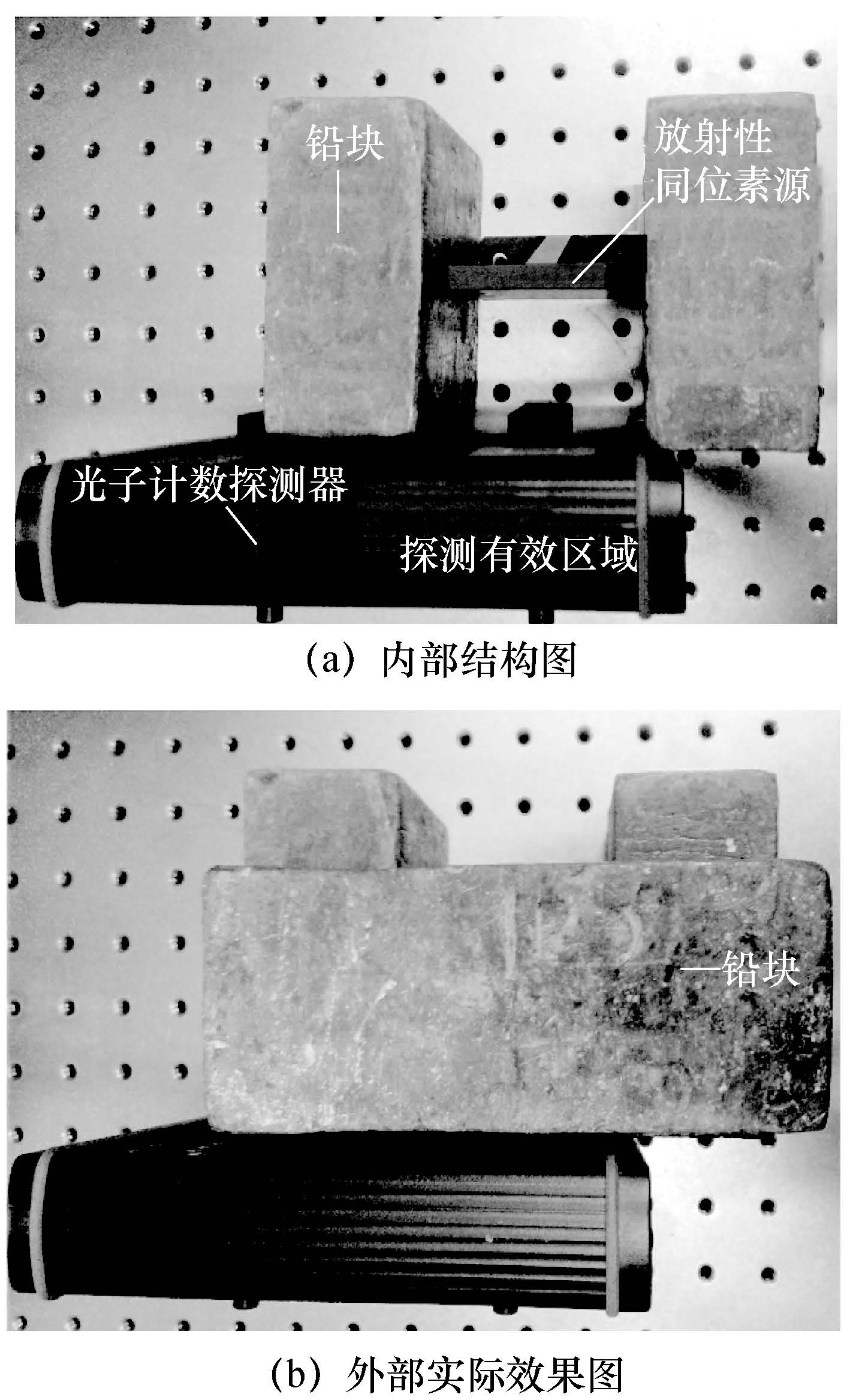图 2 光子计数探测器标定实验装置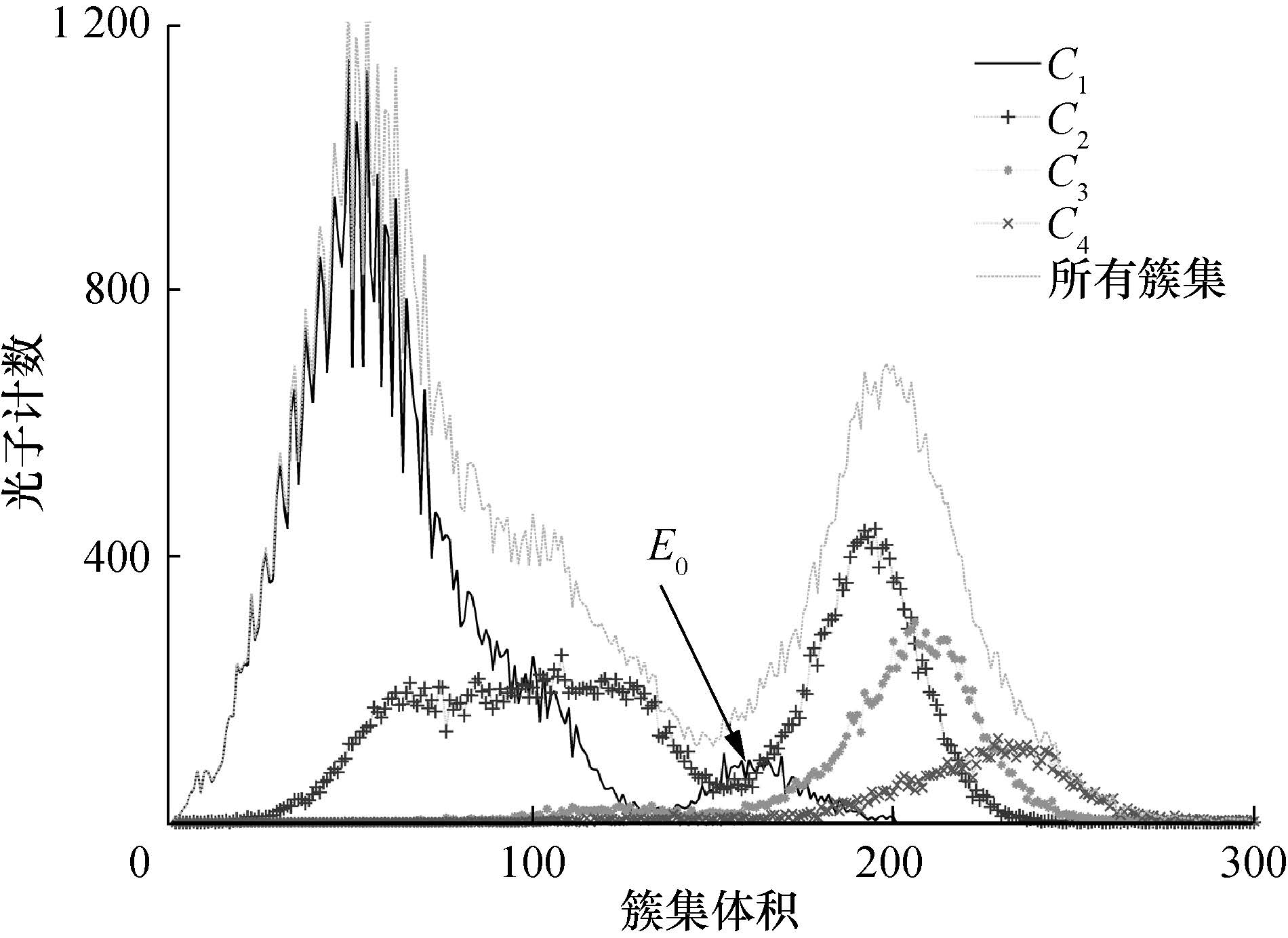图 3 未校正的单色光源241Am的TOT能谱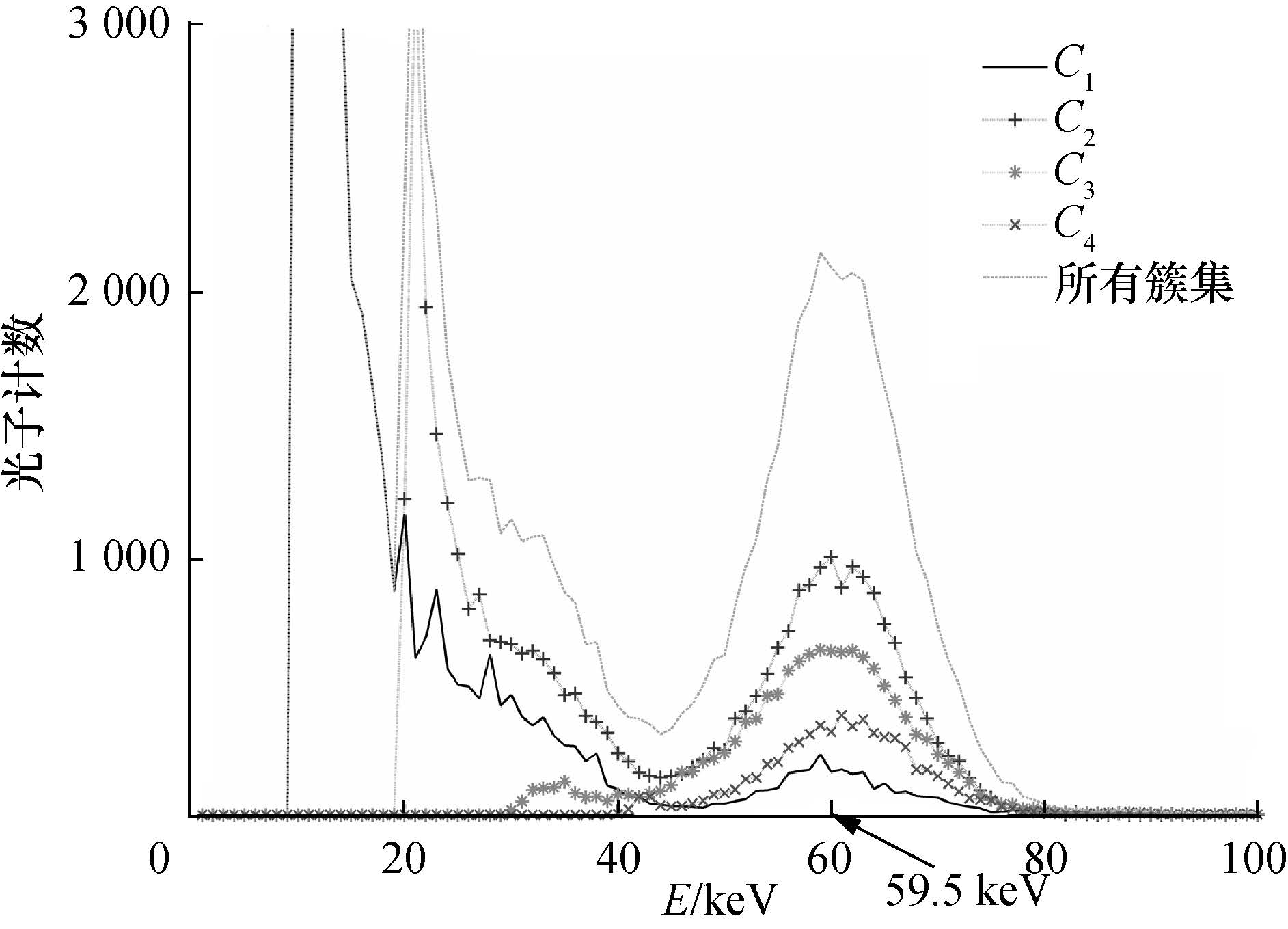图 4 校正后的放射性同位素241Am源的TOT能谱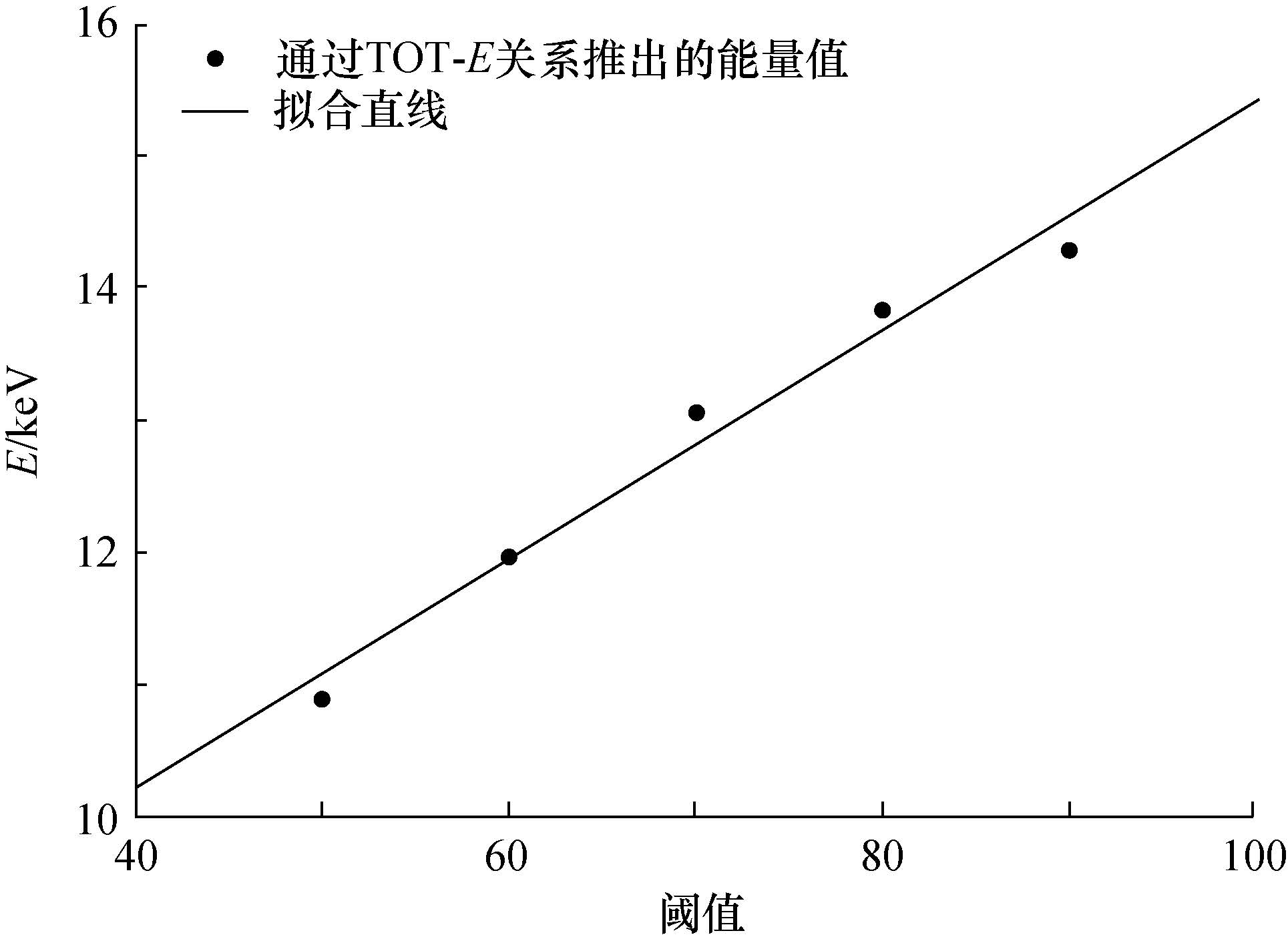图 5 光子计数探测器不同阈值[50, 90]所对应的能量图 6 未校正的放射性同位素155Eu源的TOT能谱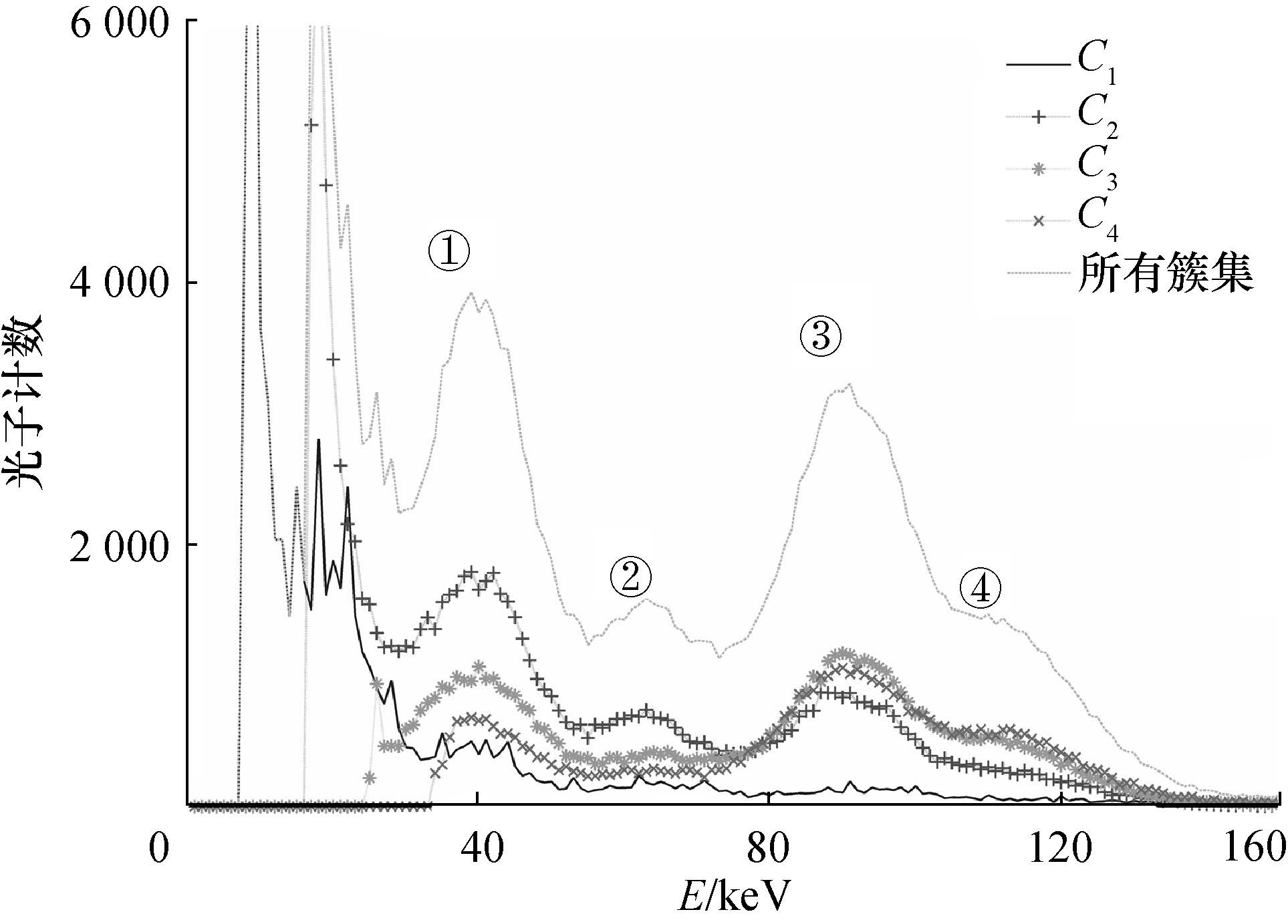图 7 校正后的放射性同位素155Eu源的TOT能谱

3 讨论与结论

  Llopart X, Campbell M, San Segundo D, et al. Medipix2, a 64k pixel read out chip with 55 μm square elements working in single photon counting mode [C]//Nuclear Science Symposium Conference Record, 2001 IEEE. San Diego, CA, USA, 2001, 3: 1484-1488.  Llopart X, Ballabriga R, Campbell M, et al. Timepix, a 65k programmable pixel readout chip for arrival time, energy and/or photon counting measurements[J]. Nuclear Instruments and Methods in Physics Research Section A: Accelerators, Spectrometers, Detectors and Associated Equipment,2007, 581 (1) : 485 –494.  Fiederle M, Greiffenberg D, Idárraga J, et al. Energy calibration measurements of MediPix2[J]. Nuclear Instruments and Methods in Physics Research Section A: Accelerators, Spectrometers, Detectors and Associated Equipment,2008, 591 (1) : 75 –79.  Pellegrini G, Chmeissani M, Maiorino M, et al. Performance limits of a 55 μm pixel CdTe detector [C]//Nuclear Science Symposium Conference Record, 2004 IEEE. Rome, Italy, 2004, 4: 2104-2109.  Ponchut C. Correction of the charge sharing in photon-counting pixel detector data[J]. Nuclear Instruments and Methods in Physics Research Section A: Accelerators, Spectrometers, Detectors and Associated Equipment,2008, 591 (1) : 311 –313.  Taguchi K, Iwanczyk J S. Vision 20/20: Single photon counting X-ray detectors in medical imaging[J]. Medical Physics,2013, 40 (10) : 100901 .  Greiffenberg D, Fauler A, Zwerger A, et al. Energy resolution and transport properties of CdTe-Timepix-Assemblies[J]. Journal of Instrumentation,2011, 6 (1) : C01058 .  Zwerger A, Fauler A, Fiederle M, et al. Medipix2: Processing and measurements of GaAs pixel detectors[J]. Nuclear Instruments and Methods in Physics Research Section A: Accelerators, Spectrometers, Detectors and Associated Equipment,2007, 576 (1) : 23 –26.  Jakubek J, Cejnarova A, Holy T, et al. Pixel detectors for imaging with heavy charged particles[J]. Nuclear Instruments and Methods in Physics Research Section A: Accelerators, Spectrometers, Detectors and Associated Equipment,2008, 591 (1) : 155 –158.  Youn H, Han J C, Kam S, et al. Energy calibration of energy-resolved photon-counting pixel detectors using laboratory polychromatic X-ray beams[J]. Nuclear Instruments and Methods in Physics Research Section A: Accelerators, Spectrometers, Detectors and Associated Equipment,2014, 760 : 79 –85.  Jakubek J. Precise energy calibration of pixel detector working in time-over-threshold mode[J]. Nuclear Instruments and Methods in Physics Research Section A: Accelerators, Spectrometers, Detectors and Associated Equipment,2011, 633 : S262 –S266.  Ponchut C, Ruat M. Energy calibration of a CdTe X-ray pixel sensor hybridized to a Timepix chip[J]. Journal of Instrumentation,2013, 8 (1) : C01005 .